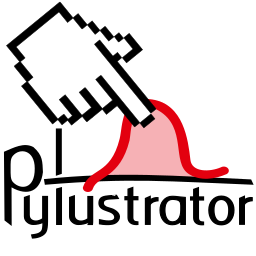# Welcome to the Pylustrator Documentation¶

Pylustrator is a software to prepare your figures for publication in a reproducible way. This means you receive a figure representing your data and alongside a generated code file that can exactly reproduce the figure as you put them in the publication, without the need to readjust things in external programs.

Pylustrator offers an interactive interface to find the best way to present your data in a figure for publication. Added formatting an styling can be saved by automatically generated code. To compose multiple figures to panels, pylustrator can compose different subfigures to a single figure.

## Installation¶

Just get pylustrator over the pip installation:

pip install pylustrator

The package depends on:

numpy, matplotlib, pyqt5, qtpy, qtawesome, scikit-image, natsort

## Usage¶

Using pylustrator is very easy and does not require substantial modifications to your code. Just add

 1 2 import pylustrator pylustrator.start() 

before creating your first figure in your code. When calling plt.show() the plot will be displayed in a pylustrator window.

You can test pylustrator with the following example code example_pylustrator.py:

  1 2 3 4 5 6 7 8 9 10 11 12 13 14 15 16 17 18 19 20 21 22 23 24 25 26 27 28 29 30 31 # import matplotlib and numpy as usual import matplotlib.pyplot as plt import numpy as np # now import pylustrator import pylustrator # activate pylustrator pylustrator.start() # build plots as you normally would np.random.seed(1) t = np.arange(0.0, 2, 0.001) y = 2 * np.sin(np.pi * t) a, b = np.random.normal(loc=(5., 3.), scale=(2., 4.), size=(100,2)).T b += a plt.figure(1) plt.subplot(131) plt.plot(t, y) plt.subplot(132) plt.plot(a, b, "o") plt.subplot(133) plt.bar(0, np.mean(a)) plt.bar(1, np.mean(b)) # show the plot in a pylustrator window plt.show() 

Saving by pressing Ctrl+S or confirming to save when closing the window will add some lines of code at the end of your python script (before your plt.show()) that defines these changes:

  1 2 3 4 5 6 7 8 9 10 #% start: automatic generated code from pylustrator plt.figure(1).set_size_inches(8.000000/2.54, 8.000000/2.54, forward=True) plt.figure(1).axes.set_position([0.191879, 0.148168, 0.798133, 0.742010]) plt.figure(1).axes.set_xlabel("data x") plt.figure(1).axes.set_ylabel("data y") plt.figure(1).axes.set_position([0.375743, 0.603616, 0.339534, 0.248372]) plt.figure(1).axes.set_xlabel("data x") plt.figure(1).axes.set_ylabel("data y") plt.figure(1).axes.set_ylim(-40.0, 90.0) #% end: automatic generated code from pylustrator 

Note

Because pylustrator can optionally save changes you’ve made in the GUI to update your source code, it cannot be used from a shell. To use pylustrator, call it directly from a python file and use the command line to execute.

The good thing is that this generated code is plain matplotlib code, so it will still work when you remove pylustrator from your code! This is especially useful if you want to distribute your code and do not want to require pylustrator as a dependency.

Can styling plots be any easier?

## Note¶

If you encounter any bugs or unexpected behaviour, you are encouraged to report a bug in our Github bugtracker.

## Citing Pylustrator¶

If you use Pylustrator for your publications I would highly appreciate it if you cite the Pylustrator:

• Gerum, R., (2020). pylustrator: code generation for reproducible figures for publication. Journal of Open Source Software, 5(51), 1989.